Wavelet Toolboxcwt

Continuous 1-D wavelet coefficients

Syntax

• ```COEFS = cwt(S,SCALES,'`wname`')
COEFS = cwt(S,SCALES,'`wname`','plot')
`COEFS = cwt(S,SCALES,`'`wname`'```,PLOTMODE)
````COEFS = cwt(S,SCALES,`'`wname`'```,PLOTMODE,XLIM)
``````

Description

`cwt` is a one-dimensional wavelet analysis function.

`COEFS = cwt(S,SCALES,`'`wname`'`)` computes the continuous wavelet coefficients of the vector `S` at real, positive `SCALES`, using the wavelet whose name is '`wname`' (see `waveinfo` for more information).

The signal S is real, the wavelet can be real or complex.

`COEFS = cwt(S,SCALES,`'`wname`'`,'plot')` computes and, in addition, plots the continuous wavelet transform coefficients.

`COEFS = cwt(S,SCALES,`'`wname`'`,PLOTMODE)` computes and plots the continuous wavelet transform coefficients.

Coefficients are colored using `PLOTMODE`. Valid values for the string `PLOTMODE `are listed in the table below.

 `PLOTMODE` Description `'lvl'` Coloration made scale-by-scale `'glb'` Coloration made considering all scales `'abslvl'` or `'lvlabs'` Coloration made scale-by-scale using the absolute values of the coefficients `'absglb'` or `'glbabs'` Coloration made considering all scales using the absolute values of the coefficients

`COEFS = cwt(...,'plot')` is equivalent to `COEFS = cwt(...,'absglb')`

 Note    You can get 3-D plots (surfaces) using the same keywords listed above for the `PLOTMODE` parameter, preceded by `'3D'`. For example: `COEFS = cwt(...,'3Dplot')`or `COEFS = cwt(...,'3Dlvl') ...`

`COEFS = cwt(S,SCALES,`'`wname`'`,PLOTMODE,XLIM)` computes and plots the continuous wavelet transform coefficients.

Coefficients are colored using `PLOTMODE` and `XLIM.`

`XLIM = `[x1 x2] with 1x1 < x2`length`(`S`)

Let s be the signal and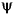the wavelet. The wavelet coefficient of s at scale a and position b is defined by

•Since s(t) is a discrete signal, we use a piecewise constant interpolation of the s(k) values, k = 1 to `length`(s).

For each given scale a within the vector `SCALES`, the wavelet coefficients Ca,b are computed for b = 1 to ls = length(s), and are stored in `COEFS`(i,:) if a = `SCALES`(i).

Output argument `COEFS` is a la-by-ls matrix where la is the length of `SCALES`. `COEFS` is a real or complex matrix depending on the wavelet type.

Examples of valid uses are

• ```t = linspace(-1,1,512);
s = 1-abs(t);
c = cwt(s,1:32,'cgau4');
c = cwt(s,[64 32 16:-2:2],'morl');
c = cwt(s,[3 18 12.9 7 1.5],'db2');
c = cwt(s,1:64,'sym4','`abslvl`',[100 400]);
```

Examples

This example demonstrates the difference between discrete and continuous wavelet transforms.

• ```% Load a fractal signal.
vonkoch=vonkoch(1:510);
lv = length(vonkoch);

subplot(311), plot(vonkoch);title('Analyzed signal.');
set(gca,'Xlim',[0 510])
% Perform discrete wavelet transform at level 5 by sym2.
% Levels 1 to 5 correspond to scales 2, 4, 8, 16 and 32.
[c,l] = wavedec(vonkoch,5,'sym2');

% Expand discrete wavelet coefficients for plot.
% Levels 1 to 5 correspond to scales 2, 4, 8, 16 and 32.
cfd = zeros(5,lv);
for k = 1:5
d = detcoef(c,l,k);
d = d(ones(1,2^k),:);
cfd(k,:) = wkeep(d(:)',lv);
end

cfd = cfd(:);
I = find(abs(cfd)<sqrt(eps));
cfd(I)=zeros(size(I));
cfd = reshape(cfd,5,lv);

% Plot discrete coefficients.
subplot(312), colormap(pink(64));
img = image(flipud(wcodemat(cfd,64,'row')));
set(get(img,'parent'),'YtickLabel',[]);
title('Discrete Transform, absolute coefficients.')
ylabel('level')

% Perform continuous wavelet transform by sym2 at all integer
% scales from 1 to 32.
subplot(313)
ccfs = cwt(vonkoch,1:32,'sym2','plot');
title('Continuous Transform, absolute coefficients.')
colormap(pink(64));
ylabel('Scale')

% Editing some graphical properties,
% the following figure is generated.```

Algorithm

••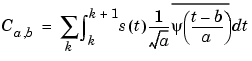if s(t) = s(k), for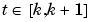then

•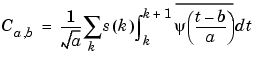•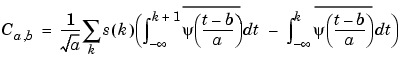So at any scale a, the wavelet coefficients Ca,b for b = 1 to `length`(s) can be obtained by convolving the signal s and a dilated and translated version of the

integrals of the form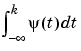(given by `intwave`), and taking the finite difference using `diff`.

```wavedec, wavefun, waveinfo, wcodemat ```coifwavf dbaux